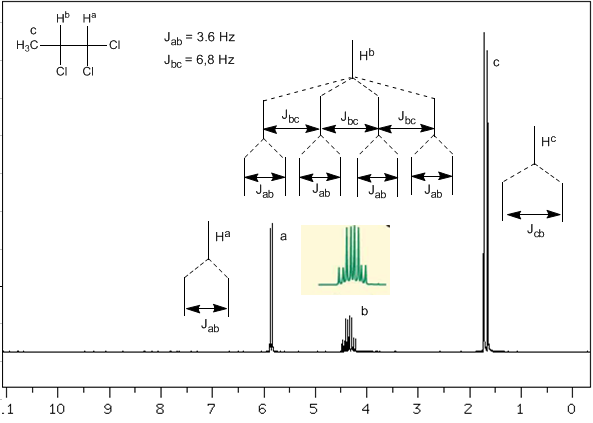Now we will analyze a more complex situation in which the nuclei $H^b$ present different coupling constants with the neighboring nuclei $H^c$ and $H^a$. Knowing that the coupling constants between these three nuclei are $J_{ab}=3.6\;Hz$ and $J_{bc}=6.8\;Hz$, to obtain the signal of $H^b$ we first couple it with $H^c$ (greatest coupling constant), giving four peaks (N+1 rule), which are then coupled with $H^a$, each splitting into two peaks. In total, a signal made up of eight peaks is obtained.